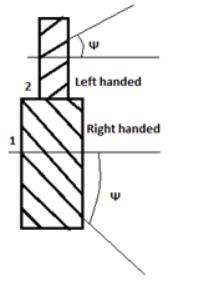Courses

# Test: Theory Of Machine- 1

## 24 Questions MCQ Test Mechanical Engineering SSC JE (Technical) | Test: Theory Of Machine- 1

Description
This mock test of Test: Theory Of Machine- 1 for Mechanical Engineering helps you for every Mechanical Engineering entrance exam. This contains 24 Multiple Choice Questions for Mechanical Engineering Test: Theory Of Machine- 1 (mcq) to study with solutions a complete question bank. The solved questions answers in this Test: Theory Of Machine- 1 quiz give you a good mix of easy questions and tough questions. Mechanical Engineering students definitely take this Test: Theory Of Machine- 1 exercise for a better result in the exam. You can find other Test: Theory Of Machine- 1 extra questions, long questions & short questions for Mechanical Engineering on EduRev as well by searching above.
QUESTION: 1

### Ball in socket joint is an example of

Solution:

The ball and socket joint is an example of spherical pair. When the two elements of a pair are connected in such a way that one element turns or swivels about the other fixed element, the pair formed is called a spherical pair.

QUESTION: 2

Solution:
QUESTION: 3

### These are 4 links joined together by a pin joint.The number of binary pairs is

Solution:
QUESTION: 4

Maximum degree of freedom of a 2D planner mechanism is,

Solution:
QUESTION: 5

Identify the given gear.Solution:

The given diagram is of a helical gear. In helical gears, the teeth are inclined to the axis of the gear. The gears can be either left handed or can be right handed depending on the direction in which the helix slopes when viewed. Here, 1 is left handed gear and 2 is a right handed gear.

QUESTION: 6

Which of the following is grubler's equation.

Solution:
QUESTION: 7

The number of inversions of a 4 bar mechanics is

Solution:

There are three inversions: 1) Beam Engine or Crank and lever mechanism. 2) Coupling rod of locomotive or double crank mechanism. 3) Watt's straight line mechanism or double lever mechanism.

QUESTION: 8

Golden linkage is a

Solution:
QUESTION: 9

Beam engine is a practical example of

Solution:
QUESTION: 10

In a crank and slotted lever quick return motion mechanism length of slotted bar in 150 cm length of crank is 50 cm and length of connecting rod is 75 cm. The stroke length is

Solution:
QUESTION: 11

If number of links is 10. The number of I. centers

Solution:
QUESTION: 12

Axode mean

Solution:
QUESTION: 13

The I-center of a sliding pair sliding at a concave upward surface lies at

Solution:
QUESTION: 14

Calculate the thrust in connecting rod, if piston effort is 200 kN and crank makes an angle of 45o from TDC. Assume obliquity ratio = 3.5

Solution:
QUESTION: 15

The acceleration respo nsible for change in direction in circular motion is

Solution:
QUESTION: 16

If velocity of slider is 4m/s and The angular velocity of the link where the slider is sliding is 5 rad/s the coriolis acceleration is

Solution:
QUESTION: 17

The direction of coriolis acceleration is

Solution:
QUESTION: 18

If M.A = Mechanical Advantage, then for a machine

Solution:
QUESTION: 19

Which of the following is a positive drive

Solution:

Positive drive means movement without slip such as the case in the linking between the crankshaft an the camshaft in the reciprocating engine. Negative drive, is an unusual term, allows slippage as with belt drive.

Gears are said to be 'POSITIVE DRIVE" because there is no slippage between the input and output.

QUESTION: 20

Which of the gears has no axial thrust

Solution:
QUESTION: 21

The velocity ratio for mitre gears is

Solution:
QUESTION: 22

For a worm and worm wheel drive
1. worm has high spiral angle
2. worm wheel has high spiral angle
3. used for very high speed reduction
4. worm wheel is the driver
Which of the following is/are correct.

Solution:

Correct Answer :- c

Explanation : The worm can be considered resembling a helical gear with a high helix angle. For extremely high helix angles, there is one continuous tooth or thread. For slightly smaller angles, there can be two, three or even more threads.

The worm wheel is similar in appearance to a spur gear the worm gear is in the form of a screw generally with a flank angle of 20°.

Self-locking means it is not possible to drive the worm using the worm wheel, and this feature is used in such things as reversing prevention systems.

QUESTION: 23

Which of the following is the most important circle of a gear.

Solution:

Correct Answer :- C

Explanation : PITCH CIRCLE is the circle derived from a number of teeth and a specified diametral or circular pitch. Circle on which spacing or tooth profiles is established and from which the tooth proportions are constructed. PITCH CYLINDER is the cylinder of diameter equal to the pitch circle.

QUESTION: 24

Diameter of a gear is 10cm and no of laths is 25.The module of the gear is

Solution: